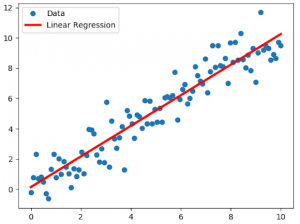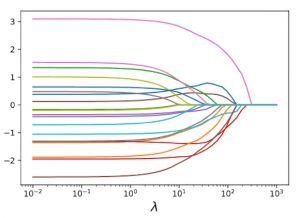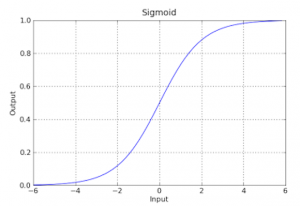# Oxagile on predictive analytics: 3 types of regression algorithms you should know

This is a guest post for the Computer Weekly Developer Network written by Yana Yelina in her role as technology writer at Oxagile, a provider of software engineering and IT consulting services.

Yelina is passionate about the ‘untapped potential’ of technology and explores the perks it can bring businesses of every colour, kind and creed.

Looking at the three common types of regression algorithms that you really should know, Yelina reminds us that if you have at least taken at least a brief foray into developing machine learning solutions, you will have heard about regression analysis.

As defined on TechTarget, logistic regression is a statistical analysis method used to predict a data value based on prior observations of a data set. A logistic regression model predicts a dependent data variable by analysing the relationship between one or more existing independent variables.

As Yelina herself puts it — in a nutshell, it’s a supervised machine learning (ML) method used to identify the causal effect relationship between a dependent and independent variable in predictive modelling.

Yelina writes from this point onwards…

Regression analysis comes in many forms, but let us briefly outline those you’ll likely need for your practice.

## #1 Linear regressionSource: quora.com

This type of statistical analysis consists of examining various data points to determine which variables are most notable predictors. Linear regression draws corresponding trend lines, such as disease outbreaks, bitcoin prices, demand for software experts, etc.

If you study the relationship between two continuous variables — with one them being an independent (explanatory, predictor) and the other one dependent (response, outcome) — you run simple regression. This type of regression analysis might take place, for example, if you need to establish the relationship between the yearly profit of an airline business (a dependent variable) and fluctuations in currency exchange rates (an independent variable).

But once you add at least one independent variable, like fuel prices or travellers’ purchasing power, this would be an example of multiple regression analysis.

## #2 Lasso regressionSource: cvxpy.org

The Lasso (Least Absolute Shrinkage and Selection Operator) is a regularisation method that analyses a collection of variables and discards those with less important coefficients.

The more variables you have, the more tedious the process becomes; and to facilitate it, the lasso automatically selects significant variables, i.e. shrinks the coefficients of unimportant predictors to zero.

Lasso regression would be a perfect fit if you plan to build a recommendation engine for an eCommerce website or a VoD platform.

## #3 Logistic regressionSource: quora.com

This is a type of an ML method utilized to predict data value based on prior observations of data sets.

Logistic regression can be:

•   Binary: the categorical response has only two possible outcomes
•   Multinomial: predicting 3+ categories (without ordering)
•      Ordinal: multiple categories are analysed and set in a particular order

Logistic regression can be applied in customer service, when you examine historical data on purchasing behaviour to personalise offerings.

## The afterword

We’ve touched upon three common models of regression analysis. When developing your predictive analysis solution, you can rely on them or try some other regression algorithms, like elastic nets, ridge regression, or stepwise model — depending on the conditions of your data. You may also need to combine regression analysis with other ML-based prediction methods.

You can reach Yana at [email protected] or connect via links shown above.

Close